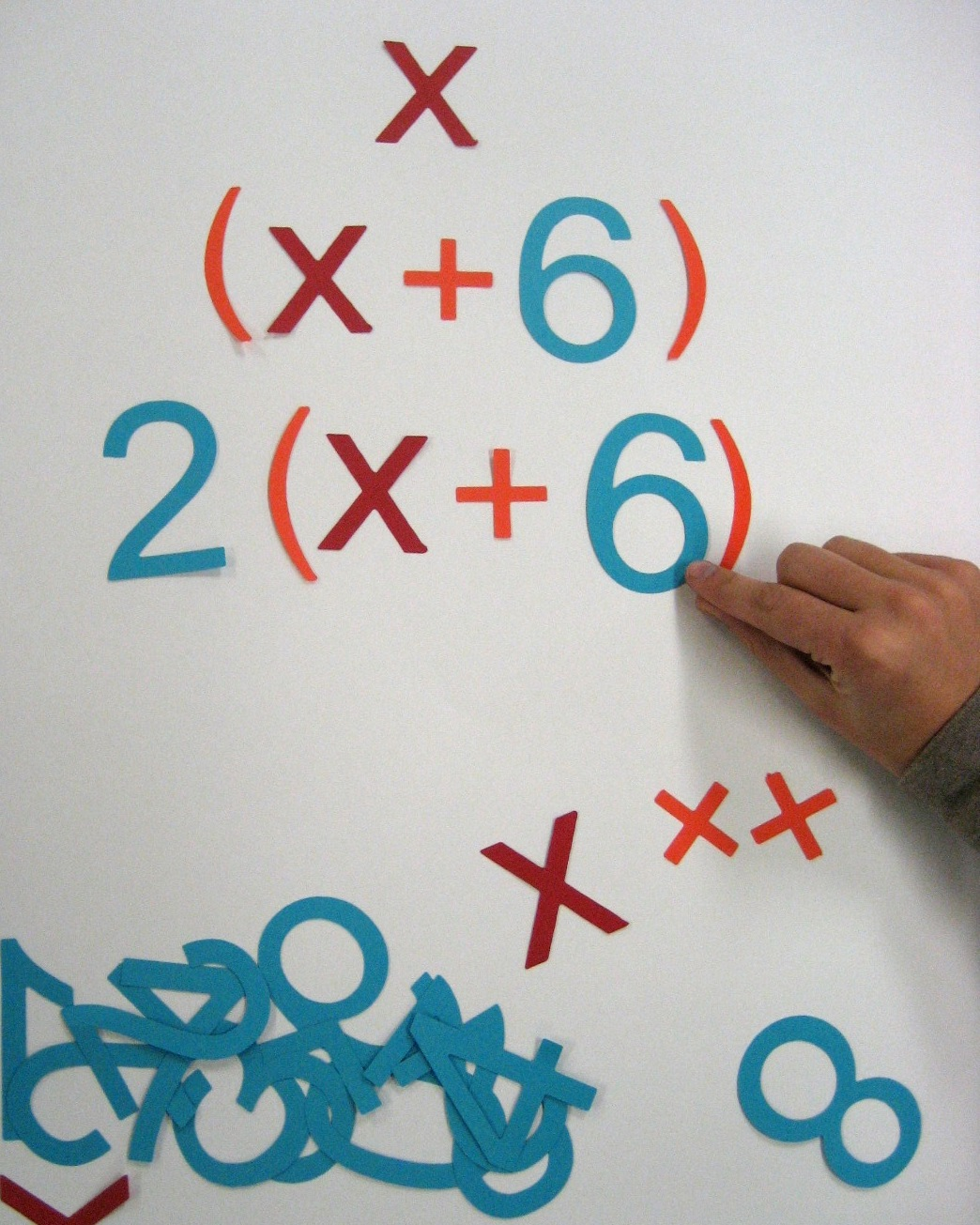{% if subscribeProperty|lower == "yes" %} {% else %} {% endif %}

By: Lindsey Graff on October 14th, 2013

Print/Save as PDF

# Grades 9-12 Activity: Number Tricks & AlgebraChallenge students to decode the algebraic equations behind number “tricks”

• “x” or a different letter used to represent the variable (cut out several)
• Numerals 0-9 (cut out 2-3 of each numeral depending on the complexity of numbers used in the number “tricks”)
• Addition, Subtraction, Multiplication and Division and Parenthesis symbols* (cut out several of each symbol)

*These symbols can also be cut using the following Cutout Maker shapes: Addition Symbol (MTH285), Subtraction Symbol (MTH286), Multiplication Symbol (MTH287), Division Symbol (MTH288), and Parentheses Symbol (MTH398).

Begin by reading a number “trick” to the class. Have students follow the instructions as you read them aloud. Tell students not to share their number with you or other students in the class.

Example:

1. Choose a number
3. Double the number
4. Subtract 8
5. Divide by 2
6. Subtract the original number
7. No matter what number you begin with, your result will always be 2!

Once students have worked through this example, as a class, walk through how to express each step of the number “trick” as an algebraic equation using the numeral and symbol cutouts.

 Instruction Algebraic Representation Choose a number x Add 6 x + 6 Double the number 2(x + 6) or 2x + 12 Subtract 8 2(x + 2) or 2x + 4 Divide by 2 x + 2 Subtract the original number 2

Then divide students into groups and give each group a number trick, a set of numeral and symbol cutouts and a poster board. Have them represent each of the operations using numerals and symbols. Once they have each operation displayed with the numerals and symbols, check their work, then have them attach the numerals and symbols to their poster and write a summary of why the “trick” works.

Have groups give a brief presentation to explain their thought process when figuring out the algebraic equations for the trick. For independent practice, have students create a “trick” of their own and prove, using algebra, that their trick will always work.